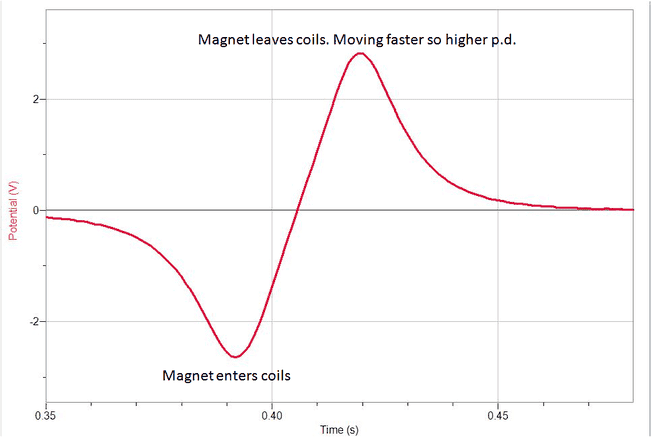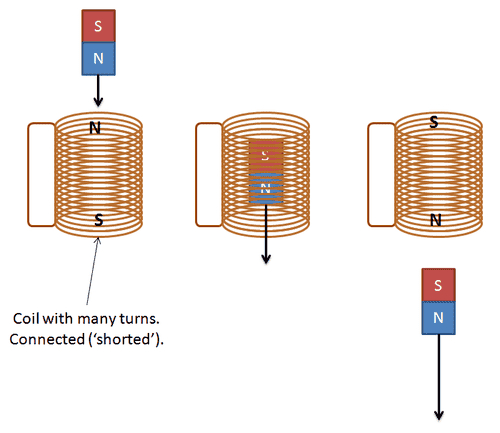# Magnetism in Coils: Why PD Changes Direction?

• Hannah7h
In summary, the graph depicted the induced emf in a coil when a magnet enters and leaves the coil. The change in direction of the emf is due to the change in magnetic flux through the coil. This change in flux induces an emf in the coil, which then produces a current in the opposite direction to oppose the change. Additionally, when the magnet enters the coil, it induces a North magnetic field in the coil, while when it leaves, it induces a South magnetic field. This is due to Lenz's law, which states that the induced current will always oppose the change that caused it. In the second case, the coil would attract the magnet, and the pole on the coil would be a North pole to oppose

#### Hannah7hThis is a graph for a magnet falling through a coil, it shows the p.d induced in the coil (the induced emf) when the magnet enters and then shows the induced emf in the coil when the magnet leaves.
My question is why does the p.d appear to change direction? (i.e why the positive and negative values?)

When the magnet is entering the coil, what is happening to the magnetic flux through the coil? What about when it is leaving the coil? What do you know about induction and its relation to the change in the magnetic flux?

Drakkith said:
When the magnet is entering the coil, what is happening to the magnetic flux through the coil? What about when it is leaving the coil? What do you know about induction and its relation to the change in the magnetic flux?

I know that an emf will be induced in the coil when there is a change in magentic flux, and that if the coil is connected in a closed circuit, a current will also be induced in the coil. I also know that the direction of this current is in such a direction to create a magnetic field which opposes the change that caused the induced current in the first place (Lenz's law). So I guess when the magnet enters the coil, there is a change in magnetic flux which induces an emf in the coil in one direction, and then when the magnet leaves the coil there is another change in flux and it induces an emf in the coil in the opposite direction, hence the positive and negative values? Is this the reason for the +ve and -ve values?

2) Also if the magnet entered the coil with its North pole, there would be a change in flux in the coil, an emf would be induced and then a current would be induced in the coil, which would generate a North magnetic field in the coil, to oppose the motion of the magnet. But I was wondering why, when the magnet leaves the coil, is another North magnetic field generated in the bottom of the coil, i.e. why do the poles on the coil switch? I have attached a picture below to try and show what I'm talking aboutIn the second case, the magnet leaves the coil. How would you have the poles on the coil in order to oppose the magnet leaving the coil? Would you have the coil attract or repel the magnet, in the third drawing?

nasu said:
In the second case, the magnet leaves the coil. How would you have the poles on the coil in order to oppose the magnet leaving the coil? Would you have the coil attract or repel the magnet, in the third drawing?
nasu said:
In the second case, the magnet leaves the coil. How would you have the poles on the coil in order to oppose the magnet leaving the coil? Would you have the coil attract or repel the magnet, in the third drawing?

You would have the coil attracting the magnet, therefore the the pole on the coil would be a north pole to attract the south pole of the magnet to oppose its motion. Ah yes I get it now, thank you

## 1. What is the phenomenon of "PD changing direction" in coils?

The phenomenon of "PD changing direction" refers to the change in polarity or direction of the induced voltage in a coil when the magnetic field passing through it is altered. This is a result of Faraday's Law of Induction, which states that a changing magnetic field will induce a voltage in a conductor.

## 2. Why does PD change direction in coils?

PD changes direction in coils due to the interaction between the magnetic field passing through the coil and the electric current flowing through it. When the magnetic field changes, it induces a voltage in the coil in the opposite direction to the current, resulting in a change in the overall direction of the induced voltage.

## 3. How does the number of coil turns affect PD changing direction?

The number of coil turns plays a significant role in the magnitude of the induced voltage and the rate at which it changes direction. More coil turns result in a stronger magnetic field and a higher induced voltage. Additionally, a larger number of coil turns will also result in a slower rate of change in the direction of the induced voltage.

## 4. Can PD change direction without a change in the magnetic field?

No, PD cannot change direction without a change in the magnetic field. As mentioned earlier, PD changing direction is a result of a changing magnetic field according to Faraday's Law of Induction. Without a change in the magnetic field, there would be no induced voltage and therefore no change in direction.

## 5. How is PD changing direction in coils used in practical applications?

The phenomenon of PD changing direction in coils is used in various practical applications, including generators, motors, and transformers. In generators, the direction of PD is constantly changing as the coil rotates in a magnetic field, producing an alternating current. In motors, the direction of the magnetic field is changed to produce motion, and in transformers, the changing magnetic field induces a voltage in a secondary coil, allowing for efficient energy transfer.